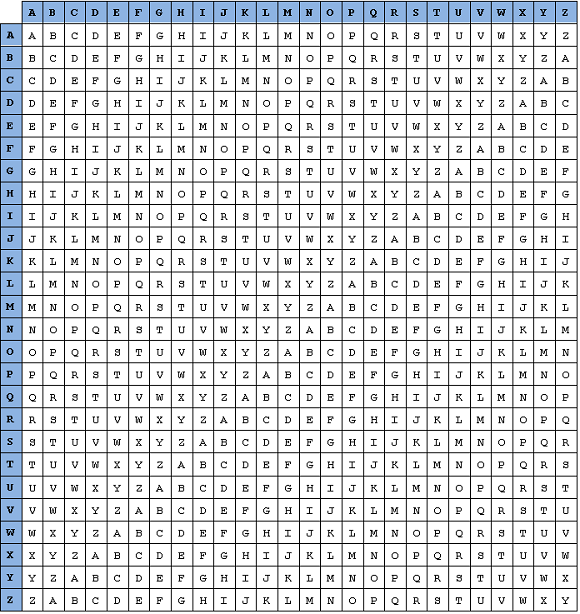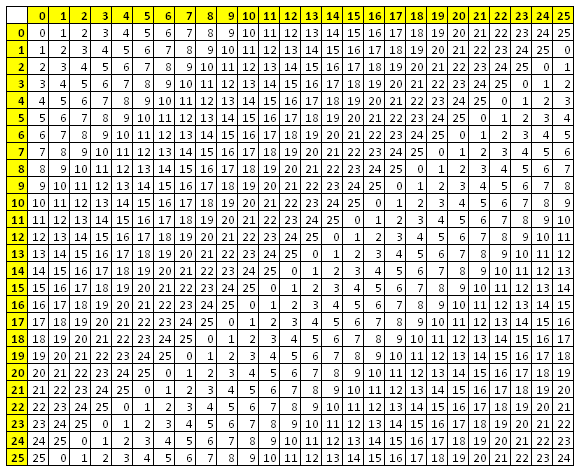# Vignère Cipher

## Introduction

The Vignère cipher is a polyalphabetic substitution cipher. That means that each plain text letter can be replaced by more than one different letter in the cipher text. It was popularised by Blaise de Vignère in the 19th century although the approach was detailed several centuries earlier. It represented a significant breakthrough in cryptography for the time and proved difficult to crack in the pre-computer era.

The basis of the cipher is a table known as the tabula recta. It is a table of cipher alphabets, 26 in total and is shown below.Notice that each of the 26 rows contains a Caesar Shift alphabet. If you number rows from 0 to 25, the shift number of each of the alphabets is equal to its row number.

To encrypt a message with Vignère, you first need to select a keyword. The letters of the keyword are used to select a cipher alphabet to use for substitution with each letter.

This example uses the keyword SECRET and the message The secret is out.

Write out the message removing all spaces and non-letter characters.

`THESECRETISOUT`

Write out the letters of the keyword underneath each letter of the message. Start from the beginning of the keyword if you run out of letters,

```THESECRETISOUT SECRETSECRETSE```

The letter in the keyword tells you which cipher alphabet to use. The first letter in the message, T will be encrypted using the alphabet in row L. The second letter of the message is encrypted using the alphabet in row E, the third with the alphabet in row M and so on.

```THESECRETISOUT SECRETSECRETSE LLGJIVJIVZWHMX```

## Programming The Vignère cipher

This is an interesting problem that seems complex at first glance but, once understood, can result in a simpler algorithm than some of the apparently less complex ciphers. The outline steps are shown here in separate stages that you can test before moving on.

### Make The Tabula Recta

Let's start by making a representation of the tabula recta and using a data structure to store it. In this case a two-dimensional array of strings or characters to store the grid. The first dimension will be the row of the cipher alphabet, the second dimension will be the column. Each value in the grid will be stored at co-ordinates represented in (row, column) format. The pseudocode assigns the letters to such an array.

The key part of this algorithm is the assignment of the letters. To see how this is done, first replace all of the letters in the tabula recta with a number from 0 to 25 depending on their position in the alphabet. You get a grid like this,We can use nested loops to produce every combination of the row and column numbers. If we can work out the number in the grid at that position, we can simply add 65 to make the ASCII value for the letter at that position.

Well, if we add the row and column numbers together and write the total in each cell, we get the following,This is nearly our target number. The problem is when the total is more than 25, we need it to 'wrap' around to 0 again. We can do that if we find the remainder (modulus) when the total is divided by 26. If we do that, we get the numbers in the first table.

```DECLARE tabula[25,25] FOR row ← 0 TO 25    FOR col ← 0 TO 25       tabula[row, col] ← CHR(65 + ((row + col) MOD 26))    END FOR END FOR```

### Clean Up The Message

The next steps are to clean up the message by removing anything that isn't a letter and make sure everything is in upper case.

```plain ← "The secret is out." upper ← UPPERCASE(plain) tidy ← "" FOR letter ← 0 TO upper.LENGTH - 1    tmpASC ← ASCII CODE OF upper[letter]    IF tmpASC > = 65 AND tmpASC <= 90 THEN       tidy ← tidy + CHR(tmpASC)    END IF END FOR plain ← tidy ```

### Encrypt Using The Keyword

When we encrypted the message by hand earlier, we wrote out the keyword many times beneath each letter. We did this all in one go to tell us which of the cipher alphabets to use. In this program we want a way of doing that for each letter as we encrypt. Again, we need to think about the numbers involved,

This is where some clear thinking can make this process easier. You need to make a loop that will visit every character in our cleaned up message. Each time we will be adding a character from the tabula array to the cipher text message. So you will be needing a line like,

`output ← output + tabula(row, col)`

The trick is knowing how to form the numbers for the row and the column. Let's use an example to light the way. In the example, the message is SHOWMETHEWAY (already cleaned up) and the keyword is LEMON.

 Message Position 0 1 2 3 4 5 6 7 8 9 10 11 Message S H O W M E T H E W A Y Col 18 7 14 22 12 4 19 7 4 22 0 24 Keyword Position 0 1 2 3 4 0 1 2 3 4 0 1 Keyword L E M O N L E M O N L E Row 11 4 12 14 13 11 4 12 14 13 11 4

The row is the cipher alphabet you will use for each character. That is determined by the letter from the keyword assigned to the plain text letter at this position. The keyword position is the remainder you get when the message position is divided by the length of the keyword. In this case, position mod 5, because the keyword is 5 characters long. You get the row number if you subtract 65 from the ASCII code of the letter at keyword(keyword position).

The col is the position of the letter you are using from the cipher alphabet. It is determined by the number of the letter at that position in the message. You can get the col number by subtracting 65 from the ASCII code of the letter at this position in the message.

So, now we need something like,

```key ← "LEMON" out ← "" FOR letter ← 0 TO plain.LENGTH - 1    out ← out + tabula[ASC(key(letter MOD key.LENGTH)) - 65, ASC(plain(letter)) - 65]    END FOR OUTPUT out```

### Tidy Up

You can do this without building the tabula at the start. The values in the tabula were based on the row and column numbers. Work on it a little and you rewrite the last block of code so that the tabula array is not used. You can also do the message tidying during the main loop.